Courses

# NCERT Solutions(Part- 4)- Comparing Quantities Class 8 Notes | EduRev

## Class 8 Mathematics by VP Classes

Created by: Full Circle

## Class 8 : NCERT Solutions(Part- 4)- Comparing Quantities Class 8 Notes | EduRev

The document NCERT Solutions(Part- 4)- Comparing Quantities Class 8 Notes | EduRev is a part of the Class 8 Course Class 8 Mathematics by VP Classes.
All you need of Class 8 at this link: Class 8

Question 1. Find CI on a sum of Rs 8000 for 2 years at 5% per annum compounded annually. Solution: We have P = Rs 8000, R = 5% p.a., T = 2 years

∵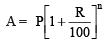∴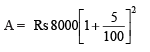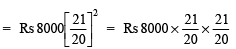= Rs (20 * 21 * 21) = Rs 8820

Now, compound interest = A – P

= Rs 8820 – Rs 8000

= Rs 820

Remember

1. The time period after which the interest is added each time to form a new principal is called the conversion period.
2. If the interest is compounded half yearly, the time period becomes twice and rate becomes half of the annual rate.
3. If the interest compounded quarterly, the time period becomes 4 times and rate become one-fourth of the annual rate.
4. If the conversion period is not specified, then it is taken as one year and the interest is compounded annually.

Question: Find the time period and rate for each.

1. A sum taken for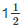years at 8% per annum is compounded half yearly.
2. A sum taken for 2 years at 4% per annum compounded half yearly.

Solution:

1. We have interest rate 8% per annum foryear.

∴ Time period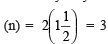half years

Rate (R) = 1/2 (8%) = 4% per half year.

2. We have interest rate 4% per annum for 2 years.

∴ Time period (n) = 2(2) = 4 half years

Rate (R) = 1/2 (4%)= 2% per half year.

Question: Find the amount to be paid

1. At the end of 2 years on Rs 2,400 at 5% per annum compounded annually.
2. At the end of 1 year on Rs 1,800 at 8% per annum compounded quarterly.

Solution:

1. We have: P = Rs 2400, R = 5% p.a., T = 2 years

∵ Interest is compounded annually i.e. n = 2

∴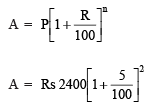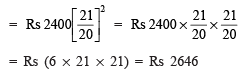2. Here, interest compounded quarterly.

∴ R = 8% p.a. = 8/4% i.e. 2% per quarter

T = 1 year = 4 * 1, i.e. 4 quarters or n = 4

Now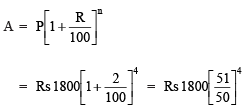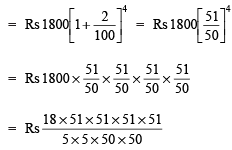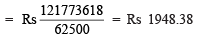Question 1. A machinery worth Rs 10,500 depreciated by 5%. Find its value after one year.

Solution: Here, P = Rs 10,500, R = –5% p.a., T = 1 year, n = 1

∵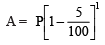[∵ Depreciation is there, ∴ r = –5%]

∴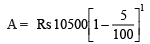= Rs10500 * 19/20 = Rs 525 * 19 = Rs 9975

Thus, machinery value after 1 year = Rs 9975

Question 2. Find the population of a city after 2 years, which is at present 12 lakh, if the rate of increase is 4%

Solution:

Present population, P = 12 lakh

Rate of increase, R = 4% p.a.

Time, T = 2 years

∴ Population after 2 years =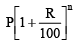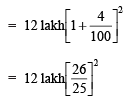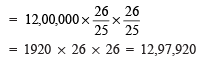Thus, the population of the town will be 12,97,920 after 2 years.

Note: For depreciation, we use the formula as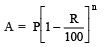Exercise 8.3

Question 1. Calculate the amount and compound interest on

(a) Rs 10,800 for 3 years at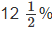per annum compounded annually.

(b) Rs 18,000 for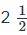years at 10% per annum compounded annually.

(c) Rs 62,500 for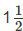years at 8% per annum compounded half yearly.

(d) Rs 8,000 for 1 year at 9% per annum compounded half yearly.

(You could use the year by year calculation using SI formula to verify.)

(e) Rs 10,000 for 1 year at 8% per annum compounded half yearly.

Solution: (a ) Here P = Rs 10800, T = 3 years, R =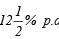We have: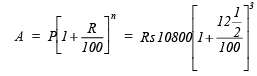[∵ Interest compounded annually,∴ n = 3]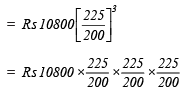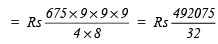∴ Amount = Rs 15377.34Rs 15377.34

Now, compound interest = Rs 15377.34 – Rs 10800

= Rs 4577.34

(b) Here P = Rs 18000, T =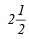years, R = 10% p.a.

∵ Interest is compounded annually,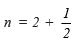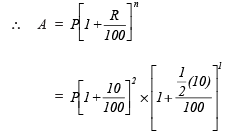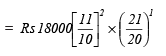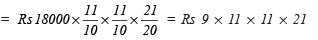= Rs 22869

∴ Amount = Rs 22869

CI = Rs 22869 – Rs 18000 = Rs 4869

(c) Here P = Rs 62500, T =r = 8% p.a.

Compounding half yearly,

R = 8% p.a. = 4% per half year

T =year → n = 3 half years.

∴ Amount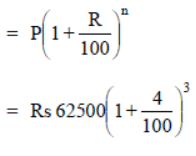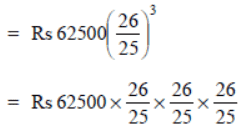= Rs 4 * 26 * 26 * 26 = Rs 70304

Amount = RS 70304

CI = Rs 70304 – Rs 62500 = Rs 7804

(d) Here P = Rs 8000, T = 1 year, R = 9% p.a.

Interest is compounded half yearly,

∴ T = 1 year = 2 half years

R = 9% p.a = 9/2% half yearly

∴ Amount =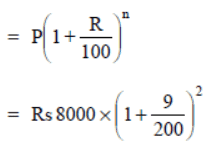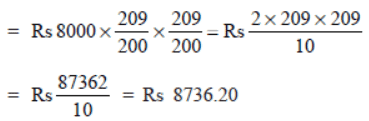CI = Rs 8736.20 – Rs 8000

= Rs 736.20

(e) Here P = Rs 10000, T = 1 year

R = 8% p.a. compounded half yearly.

∴ R = 8% p.a. = 4% per half yearly

T = 1 year → n = 2 * 1 = 2

Now, amount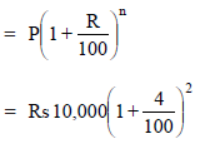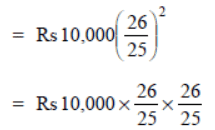= Rs 16 * 26 * 26 = Rs 10816

CI = Rs 10816 – Rs 10000 = Rs 816

Question 2. Kamala borrowed RS 26,400 from a Bank to buy a scooter at a rate of 15% p.a. compounded yearly. What amount will she pay at the end of 2 years and 4 months to clear the loan?

(Hint: Find A for 2 years with interest is compounded yearly and then find SI an the 2nd year for 4/12 years.)

Solution:

Note: Here, we shall calculate the amount for 2 years using the CI formula. Then this amount will become the principal for next 4 months, i.e. 4/12 years.

Here, P = Rs 26400, T = 2 years, R = 15% p.a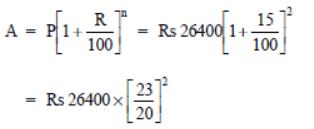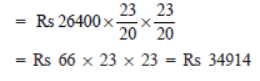Again, P = 34914, T = 4 months = 4/12 years, R = 15% p.a.

∴ Using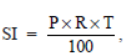we have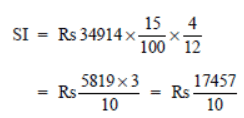= Rs 1745.70

Amount = S.I. + P

= Rs (1745.70 + 34914) = Rs 36659.70

Thus, the required amount to be paid to the bank after 2 years 4 month = Rs 36659.70

Question 3. Fabina borrows Rs 12,500 at 12% per annum for 3 years at simple interest and Radha borrows the same amount for the same time period at 10% per annum, compounded annually. Who pays more interest and by how much?

Solution:

 For FabinaP = Rs 12500 T = 3 years   R = 12% p.a.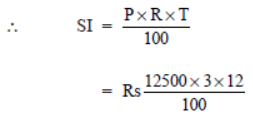= Rs 125 X 3 X 12 For RadhaP = Rs 12500T = 3 yearsR = 10% p.a. (Compounded annually)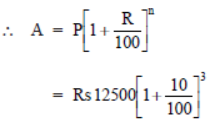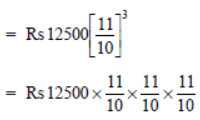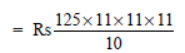= Rs 16637.5∴ CI = 16637.5 – RS 12500 = 4137.50

Difference = Rs 4500 – Rs 4137.50 = Rs 362.50

Thus, Fabina pays Rs 362.50 more.

Question 4. I borrowed Rs 12,000 from Jamshed at 6% per annum simple interest for 2 years. Had I borrowed this sum at 6% per annum compound interest, what extra amount would I have to pay?

Solution:

 For SI  Principal = Rs 12000Time = 2 years   Rate = 6% p.a.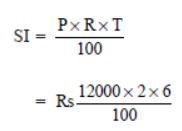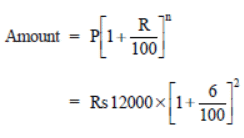For CI  Principal = Rs 12000Time = 2 years Rate = 6% p.a.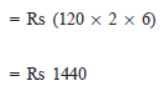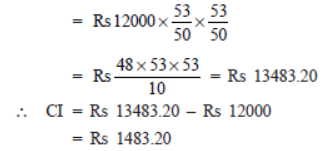Thus, excess amount = Rs 1483.20 – Rs 1440

= Rs 43.20.

Question 5. Vasudevan invested Rs 60,000 at an interest rate of 12% per annum compounded half yearly. What amount would he get

(i) after 6 months?  (ii) after 1 year?

Solution:

(i) Amount after 6 months

P = Rs 60000

T = 1/2 year , n = 1           [∵ Interest is compounded half yearly.]

R = 12% p.a. = 6% per half year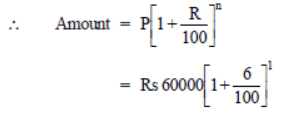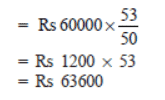(ii) Amount after 1 year

P = Rs 60000

T = 1 year; n = 2

R = 12% p.a. = 6% per half year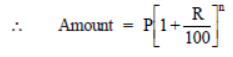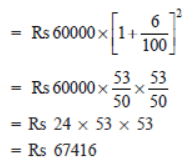Thus, amount after 6 months = Rs 63600

and amount after 1 year = Rs 67416

Question 6. Arif took a loan of Rs 80,000 from a bank. If the rate of interest is 10% per annum,find the difference in amounts he would be paying afteryears if the interest is
(i) Compounded annually. (ii) Compounded half yearly.

Solution:

(i) Compounded annually

P = Rs 80000

R = 10% p.a.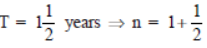Amount for 1st year.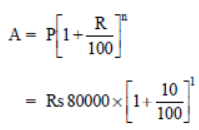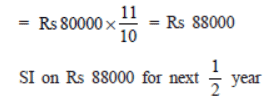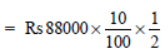= Rs 440 *10 = Rs 4400

∴ Amount = Rs 88000 + Rs 44000

= Rs 92400

(ii) Compounded half yearly

P = Rs 80000

R = 10% p.a. = 5% per half year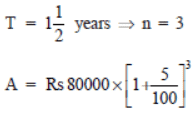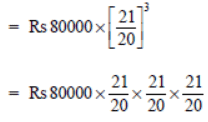= Rs 10 * 21 * 21 * 21

= Rs 92610

Thus, the difference between the two amounts = Rs 92610 – Rs 92400

= Rs 210

Question 7. Maria invested Rs 8,000 in a business. She would be paid interest at 5% per annum compounded annually. Find:
(i) The amount credited against her name at the end of the second year.
(ii) The interest for the 3rd year.

Solution: Principal = Rs 8000

Rate = 5% p.a. compounded annually

(i) Time = 2 years ⇒ n = 2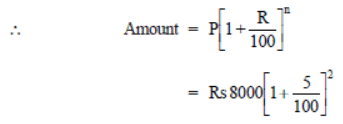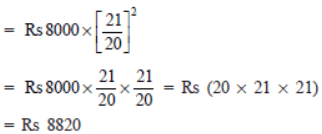∴ Amount credited against her name at the end of two years = Rs 8820

(ii) Time = 3 years ⇒ n = 3

∴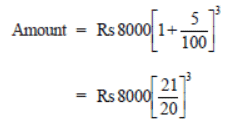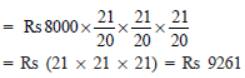= Rs (21 * 21 * 21) = Rs 9261

∵ Interest paid during 3rd year

= [Amount at the end of 3rd year] – [Amount at the end of 2nd year]

= Rs 9261 – Rs 8820 = Rs 441

Question 8. Find the amount and the compound interest on Rs 10,000 foryears at 10% per annum, compounded half yearly. Would this interest be more than the interest he would get if it was compounded annually?

Solution:

Principal = Rs 10,000

Time =%

Rate = 10% p.a.

Case I. Interest on compounded half yearly

We have r = 10 p.a. = 5% per half yearly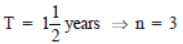∴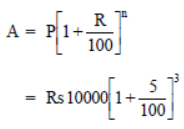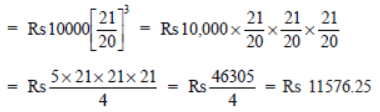∴ Amount = Rs 11576.25

Now CI = Amount – Principal

= Rs 11576.25 – Rs 10,000 = Rs 1576.25

Case II. Interest on compounded annually

We have R = 10% p.a.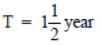Amount for 1 year =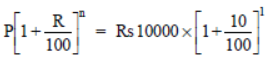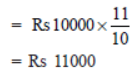∴ Interest for 1st year = Rs 11000 – Rs 10,000 = Rs 1000

Interest for next 1/2 year on Rs 11000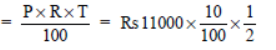= Rs 55 * 10 = Rs 550

∴ Total interest = Rs 1000 + Rs 550

= Rs 1550

Since Rs 1576.25 > Rs 1550

∴ Interest would be more in case of it is compounded half yearly.

Question 9. Find the amount which Ram will get on Rs 4096, if he gave it for 18 months at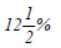per annum, interest being compounded half yearly.

Solution:

We have P = Rs 4096

T = 18 months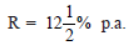∵ Interest is compounded half yearly.

T = 18 months ⇒ n = 18/6 = 3 six months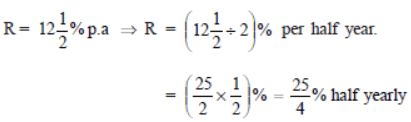Now,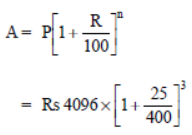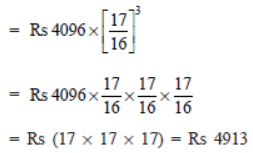Thus, the required amount = Rs 4913

Question 10. The population of a place increased to 54,000 in 2003 at a rate of 5% per annum.
(i) Find the population in 2001.
(ii) What would be its population is 2005?

Solution: Population in 2003 is P = 54000

(i) Let the population in 2001 (i.e. 2 years ago) = P

Since rate of increment in population = 5% p.a.

∴ Present population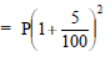or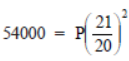or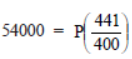or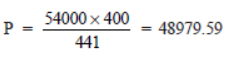= 48980 (approx.)

Thus, the population in 2001 was about 48980.

(ii) Initial population (in 2003), i.e. P = 54000

Rate of increment in population = 5% p.a.

Time = 2 years ⇒n = 2

∴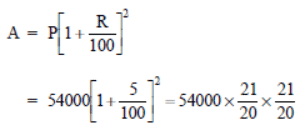= 135 * 21 * 21 = 59535

Thus, the population in 2005 = 59535.

Question 11. In a laboratory, the count of bacteria in a certain experiment was increasing at the rate of 2.5% per hour. Find the bacteria at the end of 2 hours if the count was initially 5,06,000.

Solution: Initial count of bacteria (P) = 5,06,000

Increasing rate (R) = 2.5% per hour

Time (T) = 2 hour. ⇒ n = 2

∵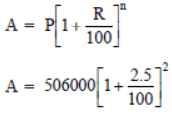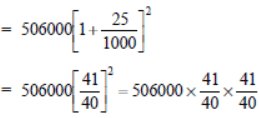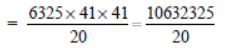= 531616.25 or 531616 (approx.)

Thus, the count of bacteria after 2 hours will be 531616 (approx.).

Question 12. A scooter was bought at Rs 42,000. Its value depreciated at the rate of 8% per annum. Find its value after one year.

Solution: Initial cost (value) of the scooter (P) = Rs 42000

Depreciation rate = 8% p.a.

Time = 1 year ⇒ n = 1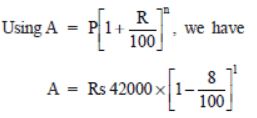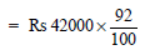= Rs 420 * 92 = Rs 38640

Thus, the value of the scooter after 1 year will be Rs 38640.

93 docs|16 tests

,

,

,

,

,

,

,

,

,

,

,

,

,

,

,

,

,

,

,

,

,

;